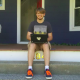author Alex McGrathTue, 28 May 2019 20:43:49 +0000 (21:43 +0100) committer David ThompsonTue, 28 May 2019 22:03:40 +0000 (18:03 -0400)
* examples/game-controller.scm: Use new sprite batch API.
* examples/grid.scm: Ditto.

 examples/game-controller.scm patch | blob | blame | history examples/grid.scm patch | blob | blame | history examples/images/controller-buttons.png patch | blob | blame | history

index 252f368..77a0ac0 100644 (file)
@@ -6,6 +6,7 @@
(chickadee render texture)
(ice-9 match))

+(define batch #f)
(define controller #f)
(define atlas #f)
(define button-icons

(define (load)
(set! no-controller-msg-pos (vec2 (center-text no-controller-msg) 240.0))
-  (set! atlas (split-texture (load-image "images/controller-buttons.png")
-                             80 80)))
+  (let ((texture (load-image "images/controller-buttons.png")))
+    (set! atlas (split-texture texture 80 80))
+    (set! batch (make-sprite-batch texture))))

(define (controller-add new-controller)
(set! controller (or controller new-controller))
(set! controller-name-pos
(vec2 (center-text (controller-name controller)) 440.0)))
-
(define (draw alpha)
(if controller
-      (with-batched-sprites
-       (draw-text (controller-name controller) controller-name-pos)
-       (for-each (match-lambda
+      (begin
+        (sprite-batch-clear! batch)
+        (draw-text (controller-name controller) controller-name-pos)
+        (for-each (match-lambda
((axis p tile)
(let ((alpha (controller-axis controller axis)))
-                      (draw-sprite (texture-atlas-ref atlas tile) p
-                                   #:tint (color 1.0 1.0 1.0 alpha)))))
+                      (sprite-batch-add!
+                       batch p
+                       #:texture-region (texture-atlas-ref atlas tile)
+                       #:tint (color 1.0 1.0 1.0 alpha)))))
trigger-icons)
(for-each (match-lambda
(((and (or 'left-stick 'right-stick) button)
(if (eq? button 'left-stick)
'left-y
'right-y))))
-                      (draw-sprite (texture-atlas-ref atlas released)
-                                   p)
+                      (sprite-batch-add! batch p
+                                         #:texture-region (texture-atlas-ref atlas released))
(when (or pressed? (not (zero? x)) (not (zero? y)))
-                        (draw-sprite (texture-atlas-ref atlas pressed)
-                                     (vec2+ p (vec2 (* x 16.0) (* y -16.0)))))))
+                        (sprite-batch-add!
+                         batch
+                         (vec2+ p (vec2 (* x 16.0) (* y -16.0)))
+                         #:texture-region (texture-atlas-ref atlas pressed)))))
((button p released pressed)
(let ((pressed? (controller-button-pressed? controller
button)))
-                      (draw-sprite (texture-atlas-ref
-                                    atlas
-                                    (if pressed? pressed released))
-                                   p))))
-                 button-icons))
+                      (sprite-batch-add!
+                       batch p
+                       #:texture-region
+                       (texture-atlas-ref atlas
+                                          (if pressed? pressed released))))))
+                 button-icons)
+       (draw-sprite-batch batch))
(draw-text no-controller-msg no-controller-msg-pos)))

(run-game #:load load #:draw draw #:controller-add controller-add)
index f20f64d..e9fe2cc 100644 (file)
(set-rect-y! %cell-rect (* y size))
(draw-filled-rect %cell-rect cell-color))
grid)
-      (with-batched-sprites
-       (for-each-cell (lambda (cell x y)
-                        (set-vec2-x! %cell-count-pos (+ (* x size) (/ size 2)))
-                        (set-vec2-y! %cell-count-pos (+ (* y size) (/ size 2)))
-                        (draw-text (number->string* (cell-count cell))
-                                   %cell-count-pos))
-                      grid)))
+      (for-each-cell (lambda (cell x y)
+                       (set-vec2-x! %cell-count-pos (+ (* x size) (/ size 2)))
+                       (set-vec2-y! %cell-count-pos (+ (* y size) (/ size 2)))
+                       (draw-text (number->string* (cell-count cell))
+                                  %cell-count-pos))
+                     grid))
(for-each-item (lambda (item rect)
(if (eq? item 'player)
(draw-filled-rect rect player-color)
index 377b397..2a4a469 100644 (file)
Binary files a/examples/images/controller-buttons.png and b/examples/images/controller-buttons.png differ